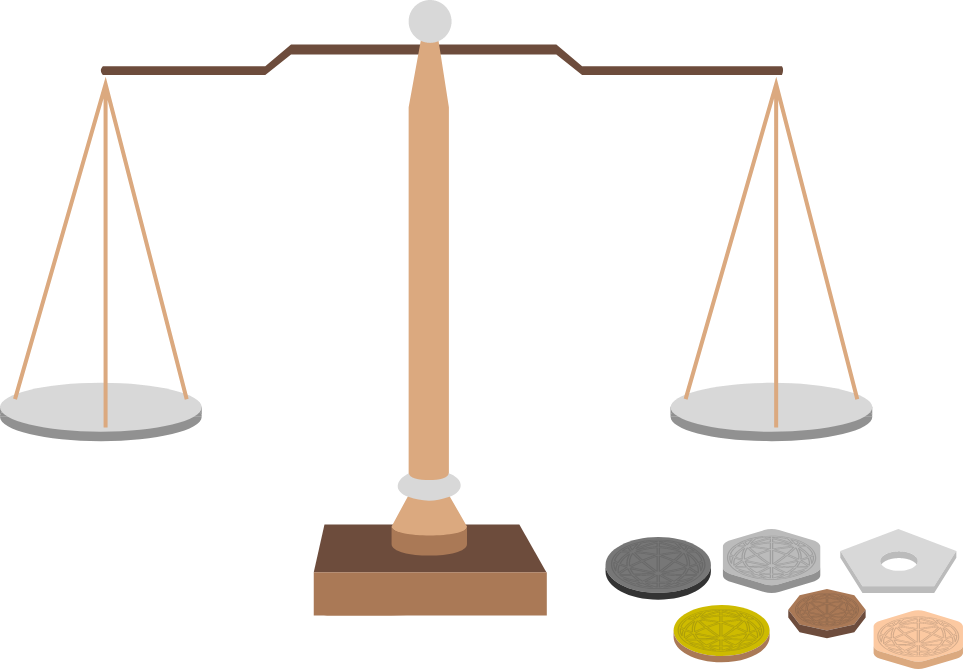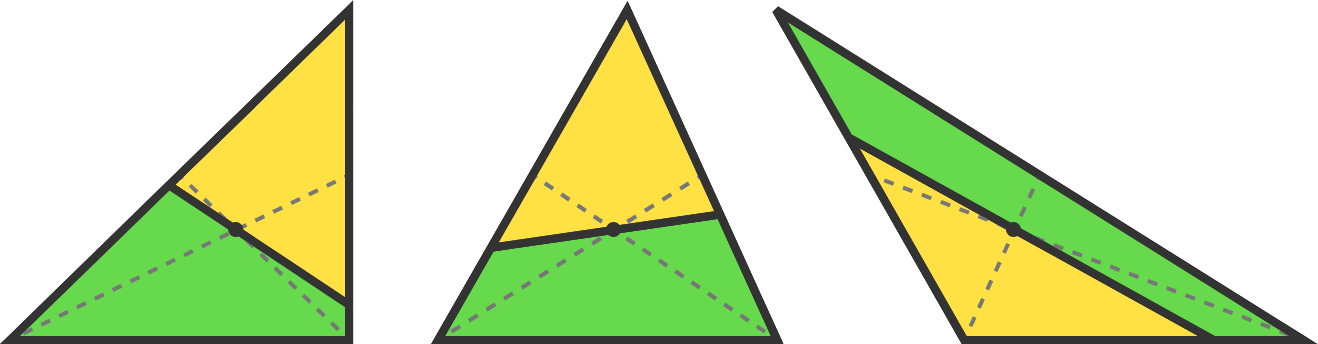# Problems of the Week

Contribute a problemYou have 6 coins of distinct weights and a beam balance with two pans. You devise an algorithm that identifies the second lightest coin with complete certainty with at most $M$ weighings.

What is the minimum possible value of $M?$

Consider trying this problem first.

A line drawn through the centroid of a triangle intersects two sides internally, forming a yellow triangle and a green quadrilateral, as shown below. What is the minimum possible ratio of the yellow area to the green area?A continuous function $f(x)$ is defined as follows for some non-zero constants $a,$ $b,$ and $c:$ f(x)=\cases{\begin{aligned} &x+a &&\text{for }|x|<2\\&bf\Big(\frac{x}{2}\Big)+c &&\text{for }|x|\ge2. \end{aligned}} Find the value of $\frac{100}{a}+\frac{100}{b}+\frac{100}{c}.$

On an infinitely large chess board, is there a configuration of kings and knights such that each king attacks exactly 2 kings and 2 knights, and each knight attacks exactly 2 kings and 2 knights?

This problem is part of the new Brilliant.org Open Problems Group (see Open Problem #1).

Call a non-empty set $\mathbb V$ of non-zero integers victorious if there exists a polynomial $P(x)$ with integer coefficients such that $P(0) = 330$ and $P(v) = 2|v|$ for all elements $v \in \mathbb V.$

How many victorious sets exist?

×